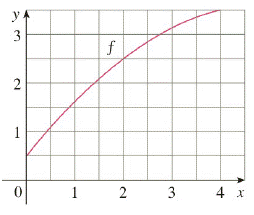Chapter 7.7, Problem 1E

Chapter
Section
Textbook Problem

# Let I = ∫ 0 4 f ( x )   d x , where f is the function whose graph is shown.(a) Use the graph to find L 2 , R 2 , and M 2 .(b) Are these underestimates or overestimates of I?(c) Use the graph to find T 2 . How does it compare with I?(d) For any value of n, list the numbers L n , R n , M n , T n , and I in increasing order.To determine

(a)

To find:

L2,R2,M2 from the given graph.

Explanation

Given:

a=0b=4n=2Δx=x(ba2)

=42=2

Formulas used:

Ln=i=1nf(xi1)Δx

Rn=i=1nf(xi)ΔxMn=Δi=1nf(x¯i)

(where xi’s are endpoints of subintervals and xi- is midpoint)

Tn=Δx2[f(x0)+2i=1n1f(xi)+f(xn)]

There are two intervals, so width of the interval is 402=2. Thus the intervals are [0,2], [2,4]

### Still sussing out bartleby?

Check out a sample textbook solution.

See a sample solution

#### The Solution to Your Study Problems

Bartleby provides explanations to thousands of textbook problems written by our experts, many with advanced degrees!

Get Started

#### Find the value of c such that the point (4, 2) lies on the graph of f(x) = cx2 + 3x 4.

Applied Calculus for the Managerial, Life, and Social Sciences: A Brief Approach

#### In Problems 19-44, factor completely. 29.

Mathematical Applications for the Management, Life, and Social Sciences

#### True or False: The graph of x = 5 is a cylinder.

Study Guide for Stewart's Multivariable Calculus, 8th

#### For what values of p does the series converge?

Study Guide for Stewart's Single Variable Calculus: Early Transcendentals, 8th

#### Explain the difference between passive and active deception.

Research Methods for the Behavioral Sciences (MindTap Course List)